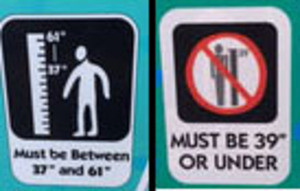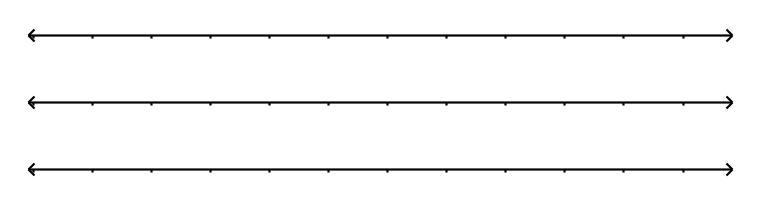# Equations and Inequalities

## Objective

Write and graph inequalities for real-world conditions. (Part 1)

## Common Core Standards

### Core Standards

?

• 6.EE.B.8 — Write an inequality of the form x > c or x < c to represent a constraint or condition in a real-world or mathematical problem. Recognize that inequalities of the form x > c or x < c have infinitely many solutions; represent solutions of such inequalities on number line diagrams.

?

• 6.NS.C.7

## Criteria for Success

?

1. Given a real-world constraint or condition, identify values that fit.
2. Write an inequality statement to represent a real-world condition.
3. Understand that a graph of an inequality represents all of the solutions, oftentimes shown as a ray.
4. Draw graphs for inequalities.

## Tips for Teachers

?

• In terms of pacing, this lesson may need to be extended over more than one day. Anchor Problem 1 includes a lot of concept development, which may require more time and practice.
• Lessons 9 and 10 engage students in using inequalities to represent constraints in real-world situations. In Lesson 9, students make the connection between the solutions to an inequality and the use of a graph to represent all of the solutions. In Lesson 10, students will interpret inequalities for different situations and distinguish between a continuous solution and a discrete solution.

#### Fishtank Plus

• Problem Set
• Student Handout Editor
• Vocabulary Package

## Anchor Problems

?

### Problem 1

The statements below describe how much money four different people have compared to $5. For each statement, • name a few values that fit the situation, • write an inequality to describe all of the solutions, and • draw a graph of the inequality on a number line. 1. Caleb has at least$5.
2. Tarek has more than $5. 3. Vanessa has at most$5.
4. Li Chen has less than \$5.

#### References

EngageNY Mathematics Grade 6 Mathematics > Module 4 > Topic H > Lesson 34Example 1

Grade 6 Mathematics > Module 4 > Topic H > Lesson 34 of the New York State Common Core Mathematics Curriculum from EngageNY and Great Minds. © 2015 Great Minds. Licensed by EngageNY of the New York State Education Department under the CC BY-NC-SA 3.0 US license. Accessed Dec. 2, 2016, 5:15 p.m..

Modified by The Match Foundation, Inc.

### Problem 2

At Sea World San Diego, kids are only allowed into the Air Bounce if they are between 37 and 61 inches tall. They are only allowed on the Tide Pool Climb if they are 39 inches tall or under:

Air Bounce                 Tide Pool Climb1. Represent the height requirements of each ride with inequalities.
2. Show the allowable heights for the rides on separate number lines.
3. Describe the height of kids who can go on the both the Air Bounce and the Tide Pool Climb.

#### References

Illustrative Mathematics Height Requirements

Height Requirements, accessed on Feb. 28, 2018, 2:51 p.m., is licensed by Illustrative Mathematics under either the CC BY 4.0 or CC BY-NC-SA 4.0. For further information, contact Illustrative Mathematics.

Modified by The Match Foundation, Inc.

### Problem 3

For each inequality below, draw a graph to represent all of the solutions to the inequality.

1. ${x>20}$2. ${2≥p}$
3. ${m>-4}$

## Problem Set

?The following resources include problems and activities aligned to the objective of the lesson that can be used to create your own problem set.

?

Write an inequality to represent each situation. Then draw a graph of the solution.

1. Kristen has a goal to walk at least 20 miles each week.1. A bookshelf can hold at most 55 books.1. A fish swims at an elevation less than 10 feet below sea level

.?